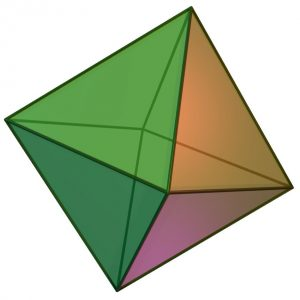# Program to calculate volume of Octahedron

• Difficulty Level : Hard
• Last Updated : 17 Mar, 2021

Given the side of the Octahedron then calculate the volume of Octahedron.
Examples:

```Input : 3
Output : 12.7279

Input : 7
Output : 161.692```

A regular Octahedron has eight faces,twelve edges and six vertices. It has eight triangles with edges of equal length and effectively two square pyramids meeting at their bases.Image Source : Wikimedia

Properties of Octahedron:
Number of faces: 8
Number of edges: 12
Number of vertices: 6
Volume = √2/3 × a3 where a is the side of Octahedron

## CPP

 `// CPP Program to calculate``// volume of Octahedron``#include ``using` `namespace` `std;` `// utility Function``double` `vol_of_octahedron(``double` `side)``{``    ``return` `((side*side*side)*(``sqrt``(2)/3));``}` `// Driver Function``int` `main()``{``    ``double` `side = 3;``    ``cout << ``"Volume of octahedron ="``         ``<< vol_of_octahedron(side)``         ``<< endl;``}`

## Java

 `// Java Program to calculate``// volume of Octahedron` `import` `java.io.*;``class` `GFG``{``    ``public` `static` `void` `main (String[] args)``      ``{``          ``// Driver Function``         ``double` `side = ``3``;``        ``System.out.print(``"Volume of octahedron = "``);``        ``System.out.println(vol_of_octahedron(side));` `      ``}` `    ``// utility Function``    ``static` `double` `vol_of_octahedron(``double` `side)``      ``{``         ``return` `((side*side*side)*(Math.sqrt(``2``)/``3``));``      ``}``}` `// This code is contributed``// by Azkia Anam.`

## Python3

 `# Python3 Program to calculate``# volume of Octahedron` `import` `math` `# utility Function``def` `vol_of_octahedron(side):``    ``return` `((side``*``side``*``side)``*``(math.sqrt(``2``)``/``3``))` `# Driver Function``side ``=` `3``print``(``"Volume of octahedron ="``,``      ``round``(vol_of_octahedron(side),``4``))` `# This code is contributed``# by Azkia Anam.`

## C#

 `// C# Program to calculate``// volume of Octahedron``using` `System;` `class` `GFG``{``    ``public` `static` `void` `Main ()``    ``{``        ``// Driver Function``        ``double` `side = 3;``        ``Console.Write(``"Volume of octahedron = "``);``        ``Console.WriteLine(vol_of_octahedron(side));` `    ``}` `    ``// utility Function``    ``static` `double` `vol_of_octahedron(``double` `side)``    ``{``        ``return` `((side*side*side)*(Math.Sqrt(2)/3));``    ``}``}` `// This code is contributed``// by vt_m.`

## PHP

 ``

## Javascript

 ``

Output:

` Volume of octahedron = 12.7279`

My Personal Notes arrow_drop_up